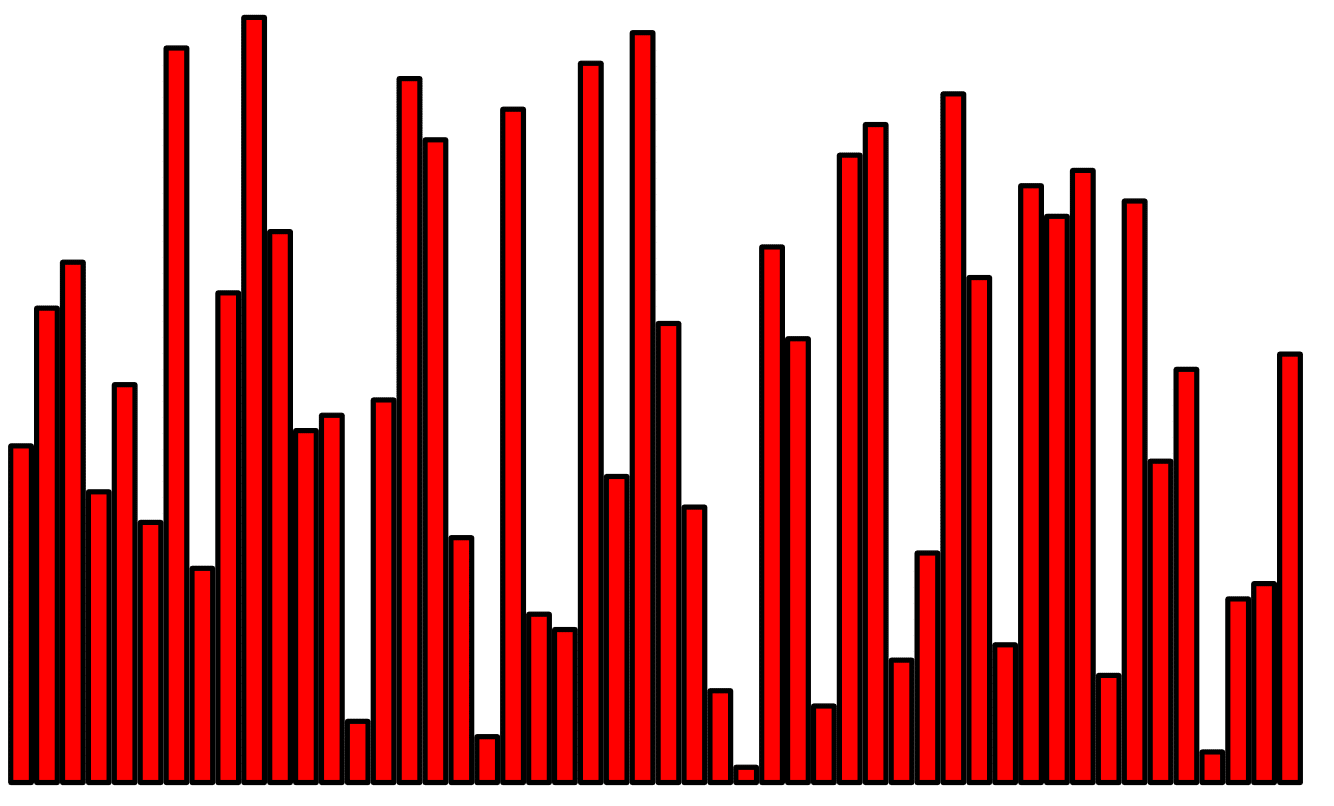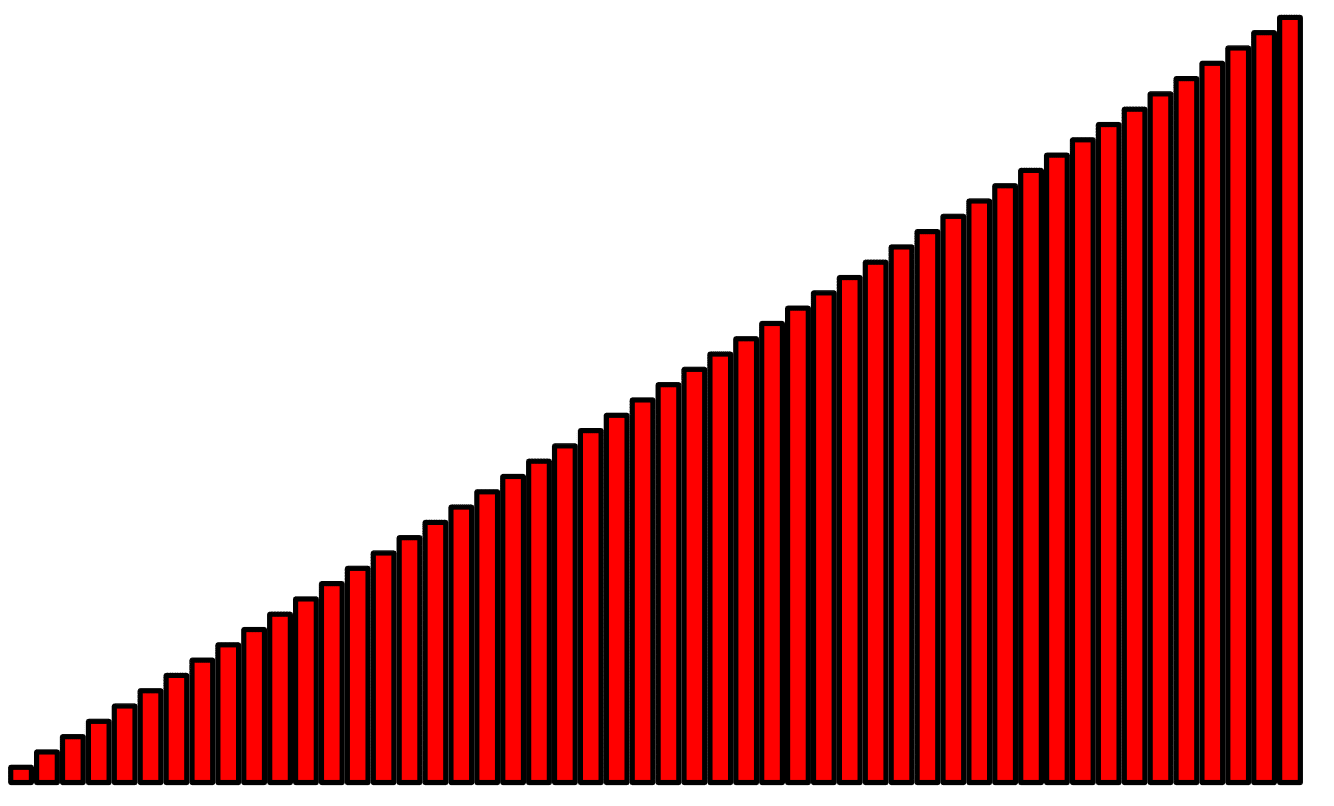# SortingInputOutput

Input Description: A set of $$n$$ items.
Problem: Arrange the items in increasing order.

Excerpt from The Algorithm Design Manual: Sorting is the fundamental algorithmic problem in computer science. Learning the different sorting algorithms is like learning scales for a musician. Sorting is the first step in solving a host of other algorithm problems. Indeed, when in doubt, sort'' is one of the first rules of algorithm design.

Sorting is also used to illustrate the standard paradigms of algorithm design. The result is that most programmers are familiar with many different sorting algorithms, which sows confusion as to which should be used for a given application.

Go To Main Page